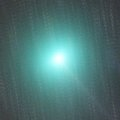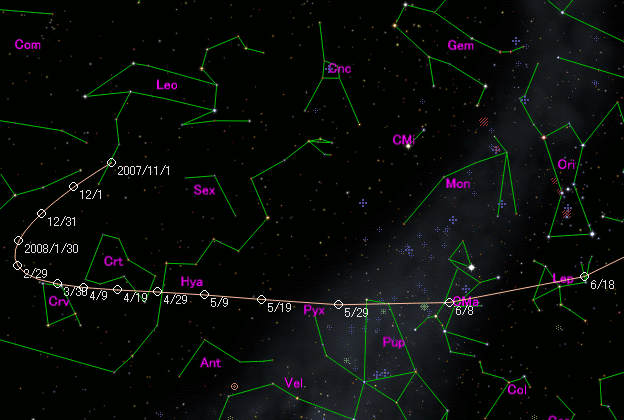# \$B%\%"%C%F%#!<%KWB@1(B

C/2007 W1 ( Boattini )###\$B%W%m%U%#!<%k(B

 \$BH/8+F|(B 2007\$BG/(B11\$B7n(B20\$BF|(B \$BH/8+8wEY(B 18.1\$BEy(B \$BH/8+ A. Boattini (Mt. Lemmon Survey)

###\$B###\$B50F;MWAG(B

```The following orbital elements are taken from MPC 63599:

Epoch 2008 June 23.0 TT = JDT 2454640.5
T 2008 June 24.8848 TT                                  MPC
q   0.849681             (2000.0)            P               Q
z  -0.000031       Peri.  306.5513      +0.1972023      +0.9775754
Node   334.5222      -0.8356367      +0.1282070
e   1.000027       Incl.    9.8889      -0.5126623      +0.1670606
From 1102 observations 2007 Nov. 20-2008 Aug. 18, mean residual 0".8.
Nongravitational parameters A1 = +3.85, A2 = -0.9414.
```

###\$B@1?^(B###\$B8wEYJQ2=(B

```        m1 = 8.5 + 5 log\$B&\$(B + 12.0 log r  [   ,130]  (              \$B!A(B2008\$BG/(B11\$B7n(B 1\$BF|(B)
m1 = 8.5 + 5 log\$B&\$(B + 25   log r  [130,   ]  (2008\$BG/(B11\$B7n(B 1\$BF|!A(B              )
```##### \$B50F;MWAG\$O(BMPC\$B\$N(BEphemerides and Orbital Elements\$B\$K7G:\\$5\$l\$?\$b\$N\$G\$9!#(B \$B@1?^\$O%9%F%i%J%S%2!<%?(B Ver.8 (\$B%"%9%H%m%"!<%D(B) \$B\$G:n@.\$7\$?\$b\$N\$G\$9!#(B \$B8wEY%0%i%U\$O(BComet for Windows\$B\$G:n@.\$7\$?\$b\$N\$G\$9!#(B